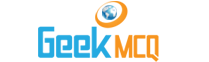# Engineering :: Thermodynamics

51.  The internal energy of a substance depdnds on
 A. Temperature B. Pressure C. Volume D. Entropy E. Velocity

52.  At critical point the enthalpy of vaporisation is
 A. Only dependent on temperature B. Zero C. Maximum D. Minimum E. None of the above

53.  The value of the product of molecular weight and the gas characteristic constant for all gases in kgf m/1 kg mol ?K is
 A. 29.27 B. 30 C. 427 D. 770 E. 848

54.  For a reversible adiabatic process the change in entropy is
 A. Zero B. Minimum C. Maximum D. Infinite E. Unity

55.  For any reversible process, the change in entropy of the system and surroundings is
 A. Zero B. Unity C. Negative D. Positive E. Infinite

56.  For any irreversible process the net entropy change is
 A. Zero B. Positive C. Negative D. Infinite E. Unity

57.  In the above problem work done is equal to
 A. 180 kcal B. 130 kcal C. 150 kcal D. 100 kcal E. 80 kcal

58.  The efficiency of cycle would be
 A. 100 percent B. 88.8 percent C. 78.3 percent D. 66.7 percent E. 37.7 percent

59.  In an isothermal process
 A. Temperature increases gradually B. Volume remains constant C. Pressure remains constant D. Enthalpy change is maximum E. Change in internal energy is zero

60.  Which of the following is incorrect?
 A. The pressure of a mixture of gases is equal to the sum of the pressure of the individual components taken each at the temperature and volume of the mixture B. The entropy of a mixture of gases is equal to the sum of the entropies of the individual components taken each at the temperature and volume of the mixture C. The internal energy of a mixture of gases is equal to the sum f the internal energies of the indicudual components taken each at the temperature and the volume of the mixture D. (B) and (C) above E. None of the above